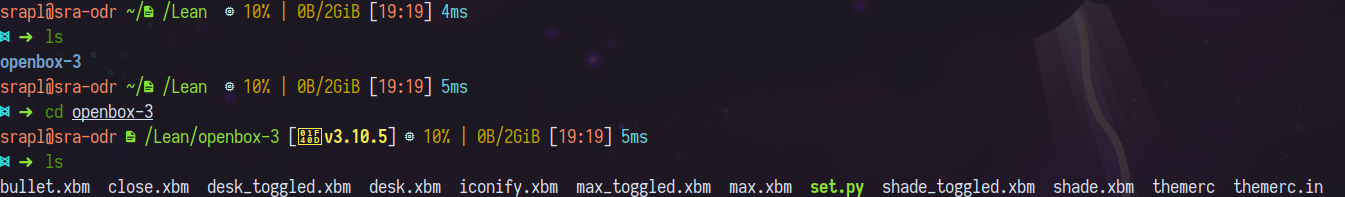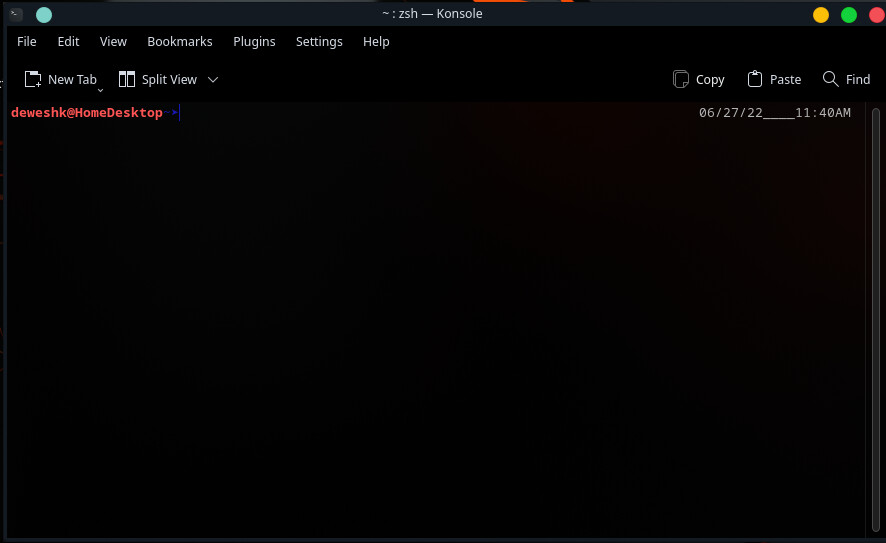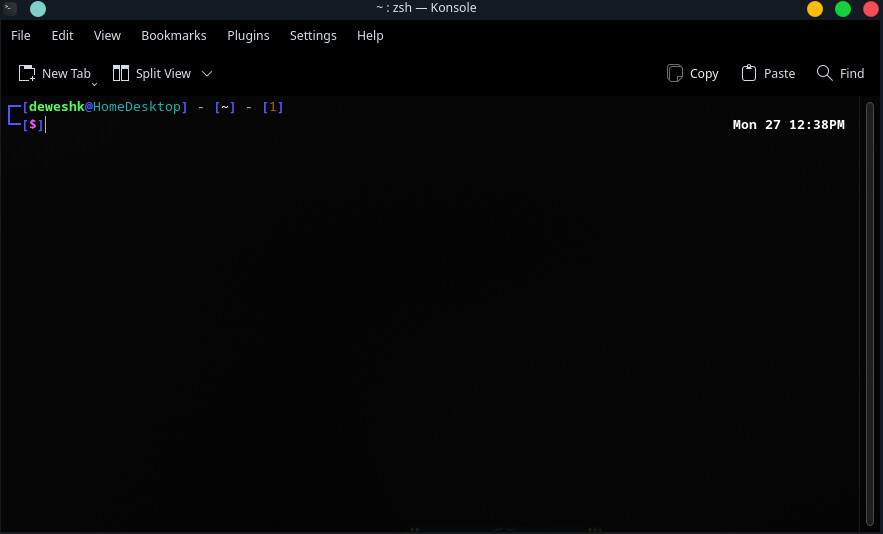# Customizing Zsh (Learning)

After some suggestions from @Kresimir, I went on my way to learn to customize prompts and theming of Zsh.

There exists ohmyzsh, which can ease up the processes, but I want to learn the way of doing it, so that I can make my own custom theme for it.

So, firstly I looked up for the default theme’s script, in order to learn the syntax used.

``````
PROMPT="%(?:%{\$fg_bold[green]%}➜ :%{\$fg_bold[red]%}➜ )"

PROMPT+=' %{\$fg[cyan]%}%c%{\$reset_color%} \$(git_prompt_info)'

ZSH_THEME_GIT_PROMPT_PREFIX="%{\$fg_bold[blue]%}git:(%{\$fg[red]%}"

ZSH_THEME_GIT_PROMPT_SUFFIX="%{\$reset_color%} "

ZSH_THEME_GIT_PROMPT_DIRTY="%{\$fg[blue]%}) %{\$fg[yellow]%}✗"

ZSH_THEME_GIT_PROMPT_CLEAN="%{\$fg[blue]%})"
``````

Also I looked up the script of better looking theme :

``````function theme_precmd {
local TERMWIDTH=\$(( COLUMNS - \${ZLE_RPROMPT_INDENT:-1} ))

PR_FILLBAR=""
PR_PWDLEN=""

local promptsize=\${#\${(%):---(%n@%m:%l)---()--}}
local rubypromptsize=\${#\${(%)\$(ruby_prompt_info)}}
local pwdsize=\${#\${(%):-%~}}

# Truncate the path if it's too long.
if (( promptsize + rubypromptsize + pwdsize > TERMWIDTH )); then
(( PR_PWDLEN = TERMWIDTH - promptsize ))
elif [[ "\${langinfo[CODESET]}" = UTF-8 ]]; then
PR_FILLBAR="\\${(l:\$(( TERMWIDTH - (promptsize + rubypromptsize + pwdsize) ))::\${PR_HBAR}:)}"
else
PR_FILLBAR="\${PR_SHIFT_IN}\\${(l:\$(( TERMWIDTH - (promptsize + rubypromptsize + pwdsize) ))::\${altchar[q]:--}:)}\${PR_SHIFT_OUT}"
fi
}

function theme_preexec {
setopt local_options extended_glob
if [[ "\$TERM" = "screen" ]]; then
local CMD=\${1[(wr)^(*=*|sudo|-*)]}
echo -n "\ek\$CMD\e\\"
fi
}

# Set the prompt

# Need this so the prompt will work.
setopt prompt_subst

# See if we can use colors.
for color in RED GREEN YELLOW BLUE MAGENTA CYAN WHITE GREY; do
typeset -g PR_\$color="%{\$terminfo[bold]\$fg[\${(L)color}]%}"
typeset -g PR_LIGHT_\$color="%{\$fg[\${(L)color}]%}"
done
PR_NO_COLOUR="%{\$terminfo[sgr0]%}"

# Modify Git prompt
ZSH_THEME_GIT_PROMPT_PREFIX=" on %{\$fg[green]%}"
ZSH_THEME_GIT_PROMPT_SUFFIX="%{\$reset_color%}"
ZSH_THEME_GIT_PROMPT_DIRTY=""
ZSH_THEME_GIT_PROMPT_CLEAN=""

ZSH_THEME_GIT_PROMPT_MODIFIED="%{\$fg[blue]%} %{%G✹%}"
ZSH_THEME_GIT_PROMPT_DELETED="%{\$fg[red]%} %{%G✖%}"
ZSH_THEME_GIT_PROMPT_RENAMED="%{\$fg[magenta]%} %{%G➜%}"
ZSH_THEME_GIT_PROMPT_UNMERGED="%{\$fg[yellow]%} %{%G═%}"
ZSH_THEME_GIT_PROMPT_UNTRACKED="%{\$fg[cyan]%} %{%G✭%}"

# Use extended characters to look nicer if supported.
if [[ "\${langinfo[CODESET]}" = UTF-8 ]]; then
PR_SET_CHARSET=""
PR_HBAR="─"
PR_ULCORNER="┌"
PR_LLCORNER="└"
PR_LRCORNER="┘"
PR_URCORNER="┐"
else
typeset -g -A altchar
set -A altchar \${(s..)terminfo[acsc]}
# Some stuff to help us draw nice lines
PR_SET_CHARSET="%{\$terminfo[enacs]%}"
PR_SHIFT_IN="%{\$terminfo[smacs]%}"
PR_SHIFT_OUT="%{\$terminfo[rmacs]%}"
PR_HBAR="\${PR_SHIFT_IN}\${altchar[q]:--}\${PR_SHIFT_OUT}"
PR_ULCORNER="\${PR_SHIFT_IN}\${altchar[l]:--}\${PR_SHIFT_OUT}"
PR_LLCORNER="\${PR_SHIFT_IN}\${altchar[m]:--}\${PR_SHIFT_OUT}"
PR_LRCORNER="\${PR_SHIFT_IN}\${altchar[j]:--}\${PR_SHIFT_OUT}"
PR_URCORNER="\${PR_SHIFT_IN}\${altchar[k]:--}\${PR_SHIFT_OUT}"
fi

# Decide if we need to set titlebar text.
case \$TERM in
xterm*)
PR_TITLEBAR=\$'%{\e]0;%(!.-=*[ROOT]*=- | .)%n@%m:%~ | \${COLUMNS}x\${LINES} | %y\a%}'
;;
screen)
PR_TITLEBAR=\$'%{\e_screen \005 (\005t) | %(!.-=[ROOT]=- | .)%n@%m:%~ | \${COLUMNS}x\${LINES} | %y\e\\%}'
;;
*)
PR_TITLEBAR=""
;;
esac

# Decide whether to set a screen title
if [[ "\$TERM" = "screen" ]]; then
PR_STITLE=\$'%{\ekzsh\e\\%}'
else
PR_STITLE=""
fi

# Finally, the prompt.
PROMPT='\${PR_SET_CHARSET}\${PR_STITLE}\${(e)PR_TITLEBAR}\
\${PR_CYAN}\${PR_ULCORNER}\${PR_HBAR}\${PR_GREY}(\
\${PR_GREEN}%\${PR_PWDLEN}<...<%~%<<\
\${PR_GREY})\$(ruby_prompt_info)\${PR_CYAN}\${PR_HBAR}\${PR_HBAR}\${(e)PR_FILLBAR}\${PR_HBAR}\${PR_GREY}(\
\${PR_CYAN}%(!.%SROOT%s.%n)\${PR_GREY}@\${PR_GREEN}%m:%l\
\${PR_GREY})\${PR_CYAN}\${PR_HBAR}\${PR_URCORNER}\
\${PR_CYAN}\${PR_LLCORNER}\${PR_BLUE}\${PR_HBAR}(\
\${PR_YELLOW}%D{%H:%M:%S}\
\${PR_LIGHT_BLUE}%{\$reset_color%}\$(git_prompt_info)\$(git_prompt_status)\${PR_BLUE})\${PR_CYAN}\${PR_HBAR}\
\${PR_HBAR}\
>\${PR_NO_COLOUR} '

# display exitcode on the right when > 0
return_code="%(?..%{\$fg[red]%}%? ↵ %{\$reset_color%})"
RPROMPT=' \$return_code\${PR_CYAN}\${PR_HBAR}\${PR_BLUE}\${PR_HBAR}\
(\${PR_YELLOW}%D{%a,%b%d}\${PR_BLUE})\${PR_HBAR}\${PR_CYAN}\${PR_LRCORNER}\${PR_NO_COLOUR}'

PS2='\${PR_CYAN}\${PR_HBAR}\
\${PR_BLUE}\${PR_HBAR}(\
\${PR_LIGHT_GREEN}%_\${PR_BLUE})\${PR_HBAR}\
\${PR_CYAN}\${PR_HBAR}\${PR_NO_COLOUR} '
``````

Now, from my side, question arises is how can I understand what are written in the scripts ?
As of now I am only aware of basic usage of conditional executions, not much more than that.
Therefore, how should I proceed ?

Depends on what you want to accomplish. If you want thorough understanding of it, this is what to read:
https://zsh.sourceforge.io/Doc/

You don’t need to read from start to finish, just be able to find what you’re interested in.

2 Likes

Analogy time:

“How can I understand what is written in Japanese*? How should I proceed?”

* or other language you don’t know

5 Likes

If you want quick solutions, look up other people’s .zshrc. Github is full of them, just search for “dotfiles”. Of course, many will contain rubbish…

Also, dig through the source code of `oh-my-zsh`, there is a bunch of nice things in there – stealing code fragments from it is the only thing this bloated monstrosity is good for, in my opinion.

Also, look on the forum, there are some useful tips for Zsh, like this one:

1 Like

Yes sir. As you had seen the recent look of the prompts in another thread, something sparked in my mind about customizing that theme.

As you told about intermixing the fragments, the problem came to my mind is about learning the effects of the parameters involved there. For this, according to me, 2 methods exists; either experimenting with the outputs of intermixing of fragments or learn the effects of various syntax.

In order to initiate the process, can I know what should be the basic beginning attributes for the prompt ?

I also found :

Edit : it’s 10:20 PM here, hence I should head for dinner, otherwise mother will begin to scold me !

3 Likes

Here is everything about prompt:

This is also a very nice, gentle introduction to Zsh (written for Mac, but most of it applies in general):

One thing I liked about KDE was this Yakuake drop down terminal, which has a oh-my-zsh look to it, and out of the box looks pretty good.

btw, to get a similar functionality, I use ddterm.

Here is mine, if you want to use it as a reference @PhoenixAwakened

2 Likes

What does the prompt look like in the command line?Prompt look is customized by starship.toml file here:

2 Likes

Looks great. I will give it it a try soon.
I have ohmyzsh running on the iMac, but yours looks way more nerdyMine is actually very simple, I can do way more fancy stuff if I wanted to.
The original theme is based on my ohmyposh theme that I made earlier. It switched to starship later.
My theme concentrates more on being simplistic, giving me only the important information and being easy on the eyes (thus the color selection)

So if you want to use ohmyposh instead of starship, you can use this:

Coming from mac (still there to some degree), ohmyzsh was my first folly into tweaking the terminal.

That’s what I want too, simplistic, with ony what I need to see.

As long as it’s dark background and not too neon colors, I am good with that.

I will take a look at it too.

Thankyou @sradjoker for sharingThanks for sharing. I will use this for understanding the various abbreviations used here.

I will go through it. Then I will try that. Afterwards I will ask you you if any issue arises.

Sir, I had started to create the file, and here is the current basic state :my doubt is; what is the character needed to use for including space between different parameters ?
As in case of RPROMPT, I unwillingly included underscore.

Space. Quote your strings.

Thanks for guiding. I had made 2 liner prompt :

``````PROMPT=\$'%{\e[0;34m%}%B┌─[%b%{\e[0m%}%{\e[1;32m%}%n%{\e[1;34m%}@%{\e[0m%}%{\e[0;36m%}%m%{\e[0;34m%}%B]%b%{\e[0m%} - %b%{\e[0;34m%}%B[%b%{\e[1;37m%}%~%{\e[0;34m%}%B]%b%{\e[0m%} - %{\e[0;34m%}%B[%b%{\e[0;33m%}%!%{\e[0;34m%}%B]%b%{\e[0m%}
%{\e[0;34m%}%B└─%B[%{\e[1;35m%}\$%{\e[0;34m%}%B]%{\e[0m%}%b'

RPROMPT=%B%F{white}%w' '%@%f%b
PS2=\$' \e[0;34m%}%B>%{\e[0m%}%b '
``````Now, what should be further reading in order to enhance it ?
As done by Sradjoker in recent posts ?

What is it that you are trying to do?

What is the problem?

The multiple color used there, and also the inclusion of special characters.
And the another thing which I want to know is, how the script makers had used “if-fi” , “elif”, etc, in their respective scripts ?

Also,

how can I read what is happening here ?